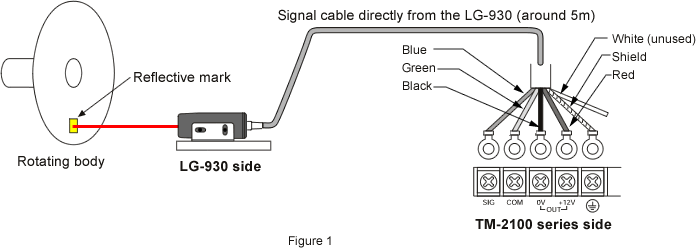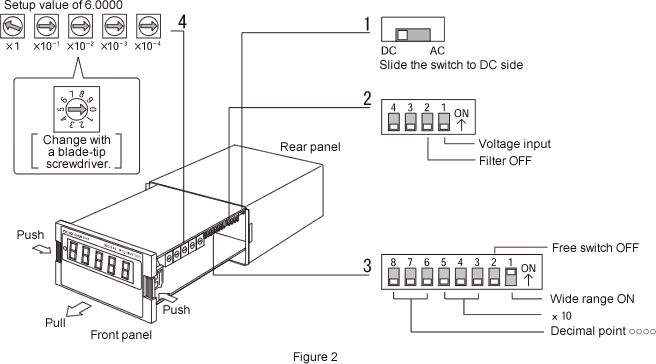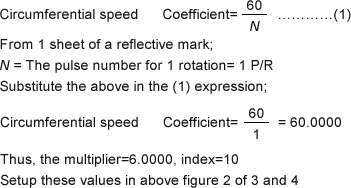Tachometer FAQSetup example for the TM-2100 series 4 "Displaying the rotation speed ○○○○ r/min after the detection from the LG-930 which sticks 1 sheet of reflective mark to the rotation axis"The setup method to display the rotation speed ○○○○ r/min after the detection of the LG-930 which sticks 1 sheet of a reflective mark to the rotating axis.

When you setup the TM-2100 series (the TM-2110, the TM-2120, the TM-2130 and the TM-2140), please pull out the body from the case as shown in the following figure 2. Please finish the setup of the TM-2100 series unit before the wiring of the power cable or signal cable.

The signal cable connection with the LG-930

Connects the signal cable which is directly connects to the LG-930 (5 m) as shown in the following figure 1. When the needs of the long cable which is more than 5 m, it is possible to make it longer with the signal cable number: D-5. Please use another connector to provide relay with the D-5 cable.Setup of the TM-2100 series

Please follow 1 to 4 steps shown in the following figure 2. This is the additional explanation of the meaning of the setup. Please refer to the instruction manual for the details of the function.

If you wish to display the [○○○○ r/min] with the LG-930 + the TM-2100 series, setup the unit with multiplier/ index [6.0000×10] and no decimal point.1. AC-DC setup

The signal from the LG-930 is square wave of 5 V in high level, so setup as [DC]. Generally, when the signal is square wave, setup as [DC] and when the signal is sine wave, setup as [AC].

2. Voltage input, filter

Because of the signal from the LG-930 is a square wave voltage, setup the 4-bit switch in the figure 2 of 2 as [bit 1: OFF (voltage input side)], [bit 2: OFF (no filter)] and [bit 3 and 4: OFF (these are free switches)]. If the signal is open collector, setup switches as [bit 1: ON (contact point input side)] and [bit 2: OFF (no filter)]. If the signal is contact point input, setup a switch as [bit 1: ON (contact point input side)] and to prevent the chattering of the contact point, setup a switch as [bit 2: ON (filter available)].

3. How to calculate the coefficient (multiplier×index)Setup the 8-bit switch of the figure 2 of 3 as [bit 3, 4 and 5: OFF] (coefficient=10). Moreover, setup switches as [bit 6, 7 and 8: OFF] (no decimal point ○○○○). Enables to setup off to third decimal point. Setup the rotary switch in the figure 2 of 4 as  (multiplier=6.0000).

4. Wide range

Setup the 8-bit switch of the figure 2 of 3 as [bit 1: ON], the frequency range becomes wide and start the measurement from the minimum measurement range of 0.1 Hz (0.1 r/min). During the stop period, it decides 0.1 Hz so you need maximum around 20 s measurement time until the display shows 0. When you setup the switch as [bit 1: OFF], the minimum measurement frequency range becomes 1 Hz (1 r/min) or more. During the stop period, you need around 1 s until the display shows 0. Also, setup the switch as [bit 2: OFF] (free switch).

Revised:2013/03/16